# npm

## echarts-stat1.2.0 • Public • Published

# ecStat

A statistical and data mining tool for Apache ECharts (incubating). You can use it to analyze data and then visualize the results with ECharts, or just use it to process data.

It works both in node.js and in the browser.

Read this in other languages: English, 简体中文.

## Installing

If you use npm, you can install it with:

## API Reference

### Histogram

A histogram is a graphical representation of the distribution of numerical data. It is an estimate of the probability distribution of a quantitative variable. It is a kind of bar graph. To construct a histogram, the first step is to "bin" the range of values - that is, divide the entire range of values into a series of intervals - and then count how many original sample values fall into each interval. The bins are usually specified as consecutive, non-overlapping intervals of a variable. Here the bins(intervals) must be adjacent, and are of equal size.

#### Syntax

• Used as echarts transform (since echarts 5.0)
• Standalone
##### Parameter
• `data` - `number[] | number[][]`. Data samples of numbers.

or

• `config` - `object`.

• `config.method` - `'squareRoot' | 'scott' | 'freedmanDiaconis' | 'sturges'`. Optional. Methods to calculate the number of bin. There is no "best" number of bin, and different bin size can reveal different feature of data.

• `squareRoot` - This is the default method, which is also used by Excel histogram. Returns the number of bin according to Square-root choice:

• `scott` - Returns the number of bin according to Scott's normal reference Rule:

• `freedmanDiaconis` - Returns the number of bin according to The Freedman-Diaconis rule:

• `sturges` - Returns the number of bin according to Sturges' formula:

• `config.dimensions` - `(number | string)`. Optional. Specify the dimensions of data that are used to regression calculation. By default `0`, which means the column 0 and 1 is used in the regression calculation. In echarts transform usage, both dimension name (`string`) and dimension index (`number`) can be specified. In standalone usage, only dimension index can be specified (not able to define dimension name).

##### Return Value (only for standalone usage)
• Used as echarts transform (since echarts 5.0)
• Standalone
• `result` - `object`. Contain detailed messages of each bin and data used for ECharts to draw the histogram.
• `result.bins` - `BinItem[]`. An array of bins, where each bin is an object (`BinItem`), containing three attributes:
• `x0` - `number`. The lower bound of the bin (inclusive).
• `x1` - `number`. The upper bound of the bin (exclusive).
• `sample` - `number[]`. Containing the associated elements from the input data.
• `result.data` - `[MeanOfV0V1, VCount, V0, V1, DisplayableName][]`. Used for bar chart to draw the histogram, where the length of `sample` is the number of sample values in this bin. For example:
• `result.customData` - `[V0, V1, VCount][]`. Used for custom chart to draw the histogram, where the length of `sample` is the number of sample values in this bin.

#### Examples

test/transform/histogram_bar.html

test/standalone/histogram_bar.html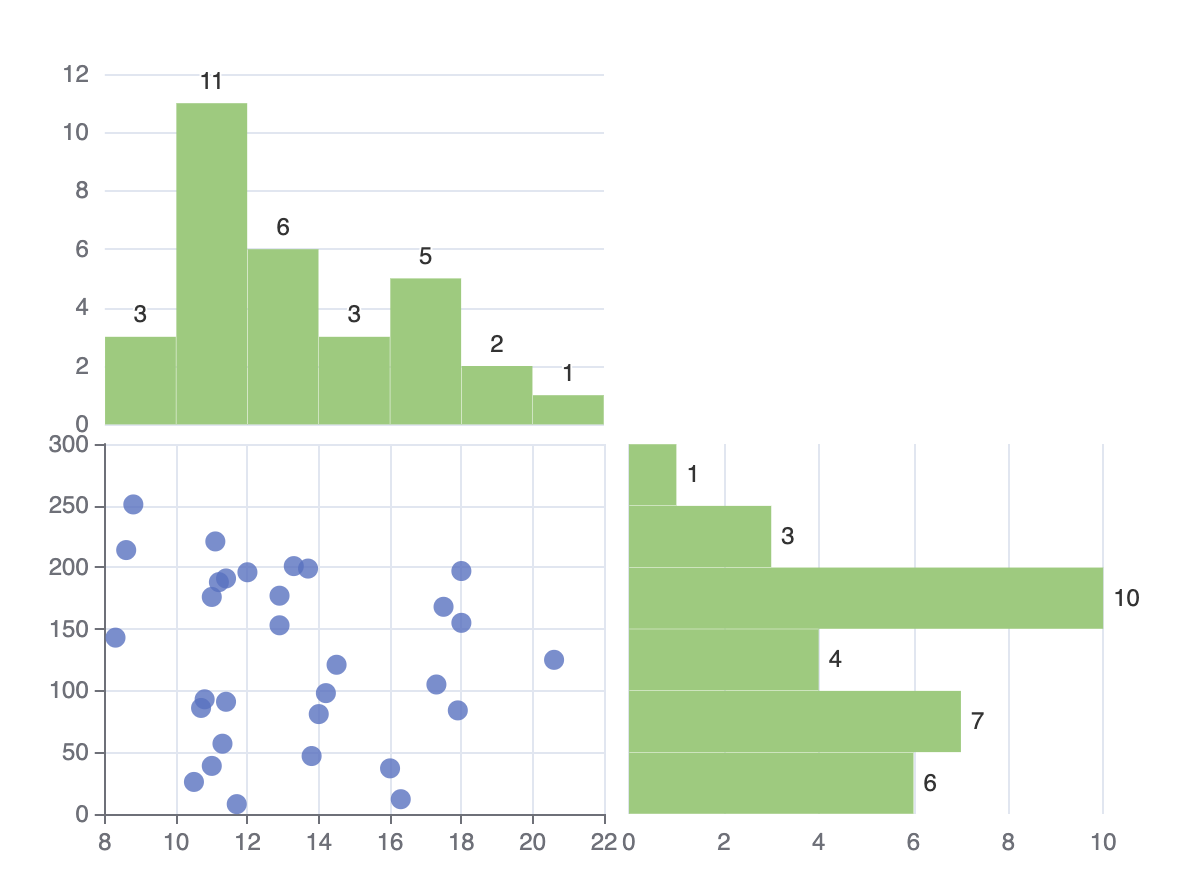Run

### Clustering

Clustering can divide the original data set into multiple data clusters with different characteristics. And through ECharts, you can visualize the results of clustering, or visualize the process of clustering.

#### Syntax

• Used as echarts transform (since echarts 5.0)
• Standalone
##### Parameter
• `data``number[][]`. Two-dimensional numeric array, each data point can have more than two numeric attributes in the original data set. In the following example, `data` is called `data point` and `data` is one of the numeric attributes of `data`.

• `config` - `object`.

• `config.clusterCount``number`. Mandatory. The number of clusters generated. Note that it must be greater than 1.
• `config.dimensions` - `(number | string)[]`. Optional. Specify which dimensions (columns) of data will be used to clustering calculation. The other columns will also be kept in the output data. By default all of the columns of the data will be used as dimensions. In echarts transform usage, both dimension name (`string`) and dimension index (`number`) can be specified. In standalone usage, only dimension index can be specified (not able to define dimension name).
• `config.stepByStep``boolean`. Optional. Control whether doing the clustering step by step. By default `false`.
• `config.outputType` - `'single' | 'multiple'`. Optional. Specify the format of the output. In "standalone" usage, it is by default `'multiple'`. In "transform" usage, it can not be specified, always be `'single'`.
• `config.outputClusterIndexDimension` - `(number | {index: number, name?: string})`. Mandatory. It only works in `config.outputType: 'single'`. In this mode, the cluster index will be written to that dimension index of the output data. If be a `number`, it means dimension index. Dimension index is mandatory, while dimension name is optional, which only enables the downstream refer this dimension by name.
• `config.outputCentroidDimensions` - `(number | {index: number, name?: string})[]` Optional. It only works in `config.outputType: 'single'`. If specify, the cluster centroid will be written to those dimensions of the result data. By default the centroids will not be written to the result data. If be a `number`, it means dimension index. Dimension index is mandatory, while dimension name is optional, which only enables the downstream refer this dimension by name.
##### Return Value

For example, the input data is:

And we specify the `config` as:

The result will be:

• Used as echarts transform (since echarts 5.0)
• Standalone
• `outputType: 'single'`:
• `result` - `object`. For example:
• `outputType: 'multiple'`:
• `result``object`. Including the centroids, and pointsInCluster. For example:

#### Examples

You can not only do cluster analysis through this interface, but also use ECharts to visualize the results.

Note: the clustering algorithm can handle multiple numeric attributes, but for the convenience of visualization, two numeric attributes are chosen here as an example.

##### Directly visualize the final results of clustering

test/transform/clustering_bikmeans.html

test/standalone/clustering_bikmeans.html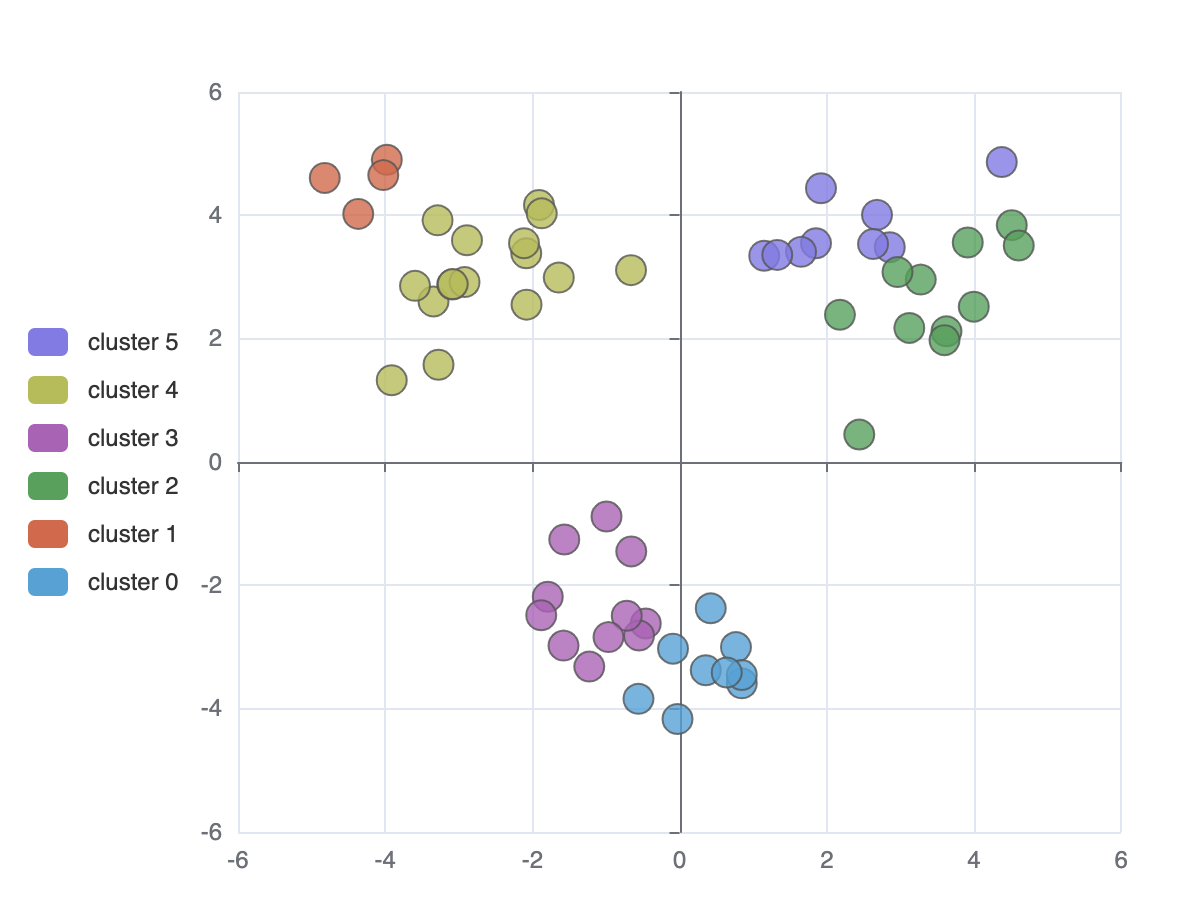Run

##### Visualize the process of clustering

test/standalone/clustering_animation.htmlRun

### Regression

Regression algorithm can according to the value of the dependent and independent variables of the data set, fitting out a curve to reflect their trends. The regression algorithm here only supports two numeric attributes.

#### Syntax

• Used as echarts transform (since echarts 5.0)
• Standalone
##### Parameters
• `regressionType` - `string`. Mandatory. There are four types of regression, which are `'linear'`, `'exponential'`, `'logarithmic'`, `'polynomial'`.
• `data` - `number[][]`. Two-dimensional numeric array, Each data object should have two numeric attributes in the original data set. For Example:
• `opt` - `object`.
• `opt.dimensions` - `(number | string)[] | (number | string)`. Optional. Specify the dimensions of data that are used to regression calculation. By default `[0, 1]`, which means the column 0 and 1 is used in the regression calculation. In echarts transform usage, both dimension name (`string`) and dimension index (`number`) can be specified. In standalone usage, only dimension index can be specified (not able to define dimension name).
• `opt.order` - `number`. Optional. By default `2`. The order of polynomial. If you choose other types of regression, you can ignore it.
##### Return Value (only for standalone usage)
• Used as echarts transform (since echarts 5.0)
• Standalone
• `myRegression` - `object`. Including points, parameter, and expression. For Example:

#### Examples

You can not only do regression analysis through this interface, you can also use ECharts to visualize the results.

##### Linear Regression

test/transform/regression_linear.html

test/standalone/regression_linear.html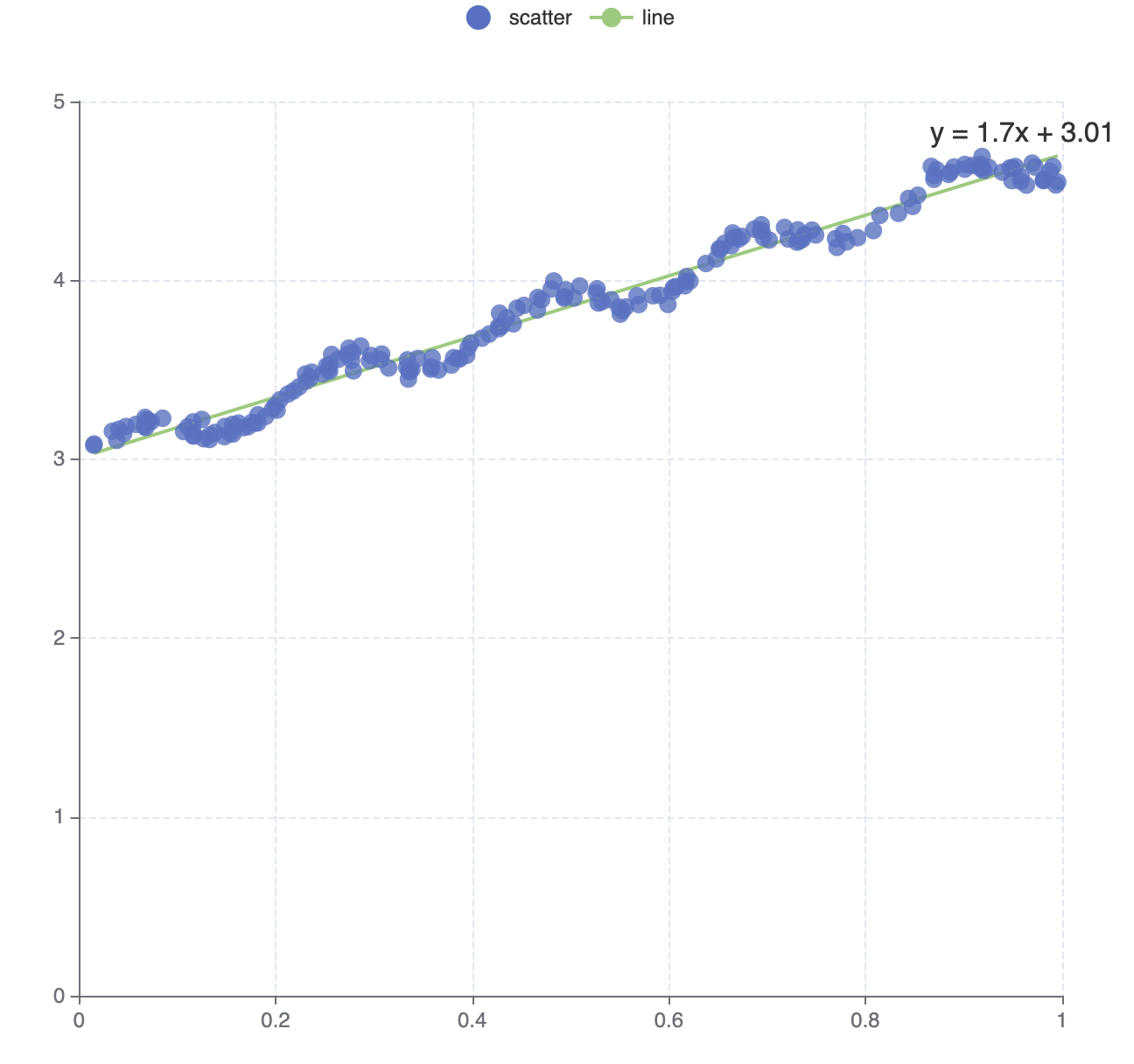Run

##### Exponential Regression

test/transform/regression_exponential.html

test/standalone/regression_exponential.html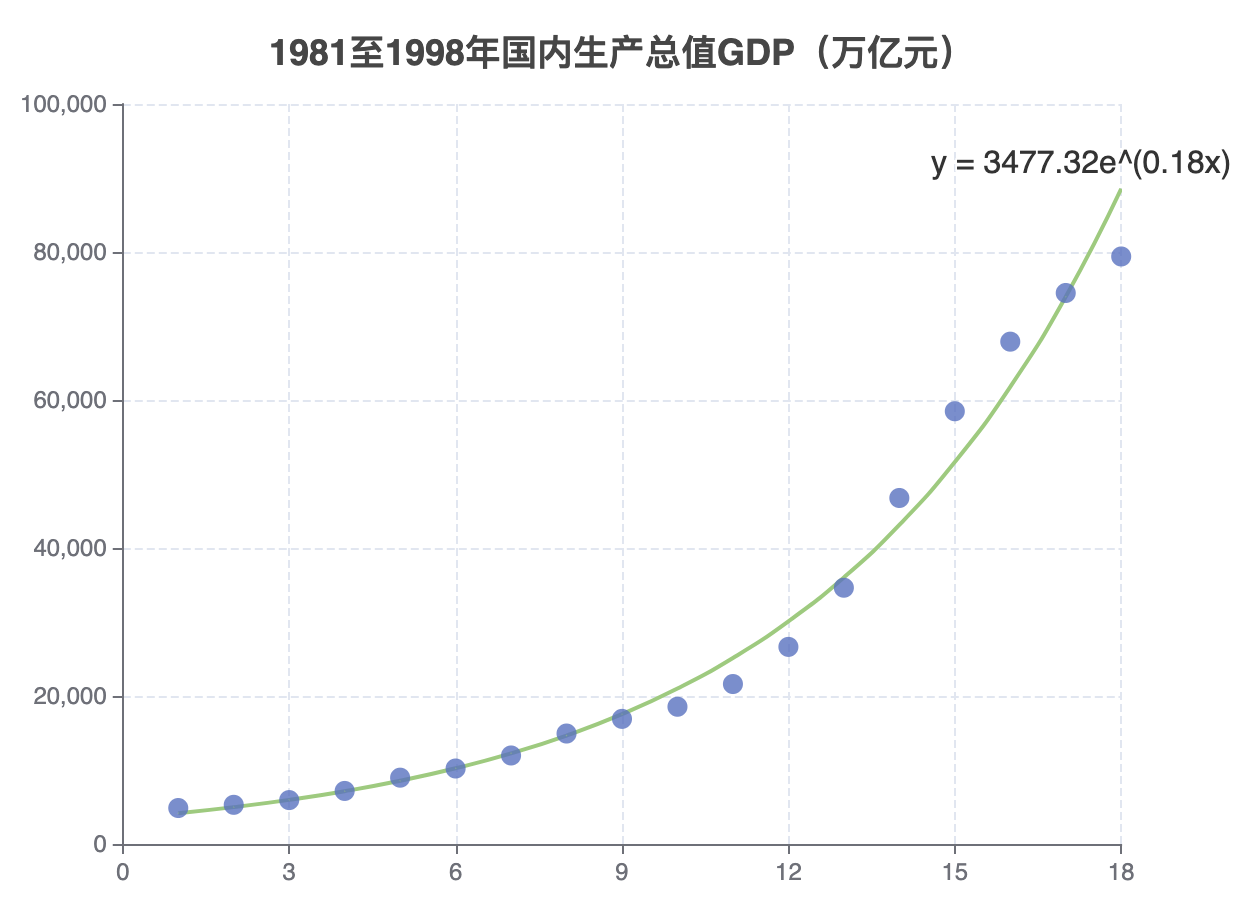Run

##### Logarithmic Regression

test/transform/regression_logarithmic.html

test/standalone/regression_logarithmic.html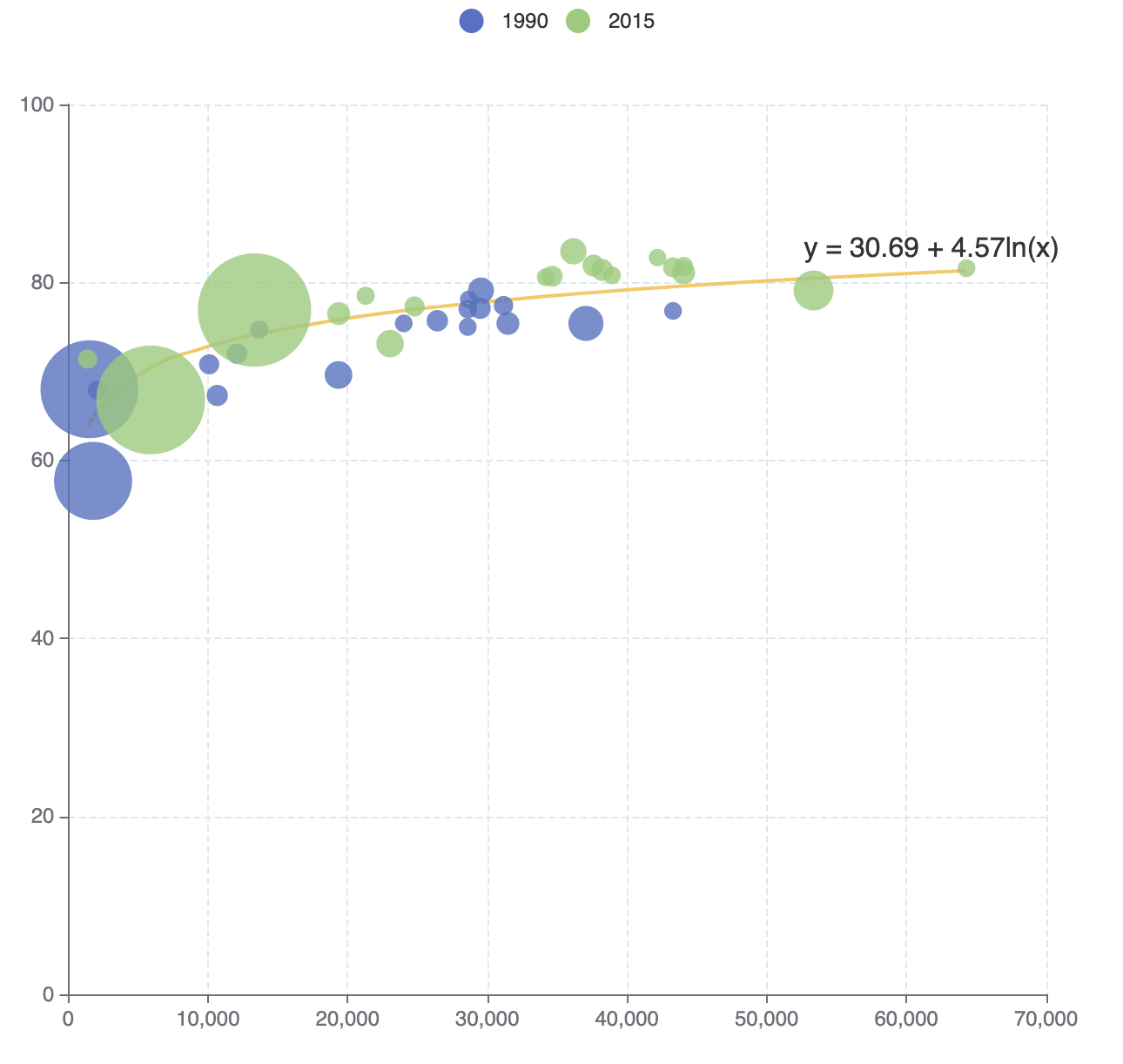Run

##### Polynomial Regression

test/transform/regression_polynomial.html

test/standalone/regression_polynomial.html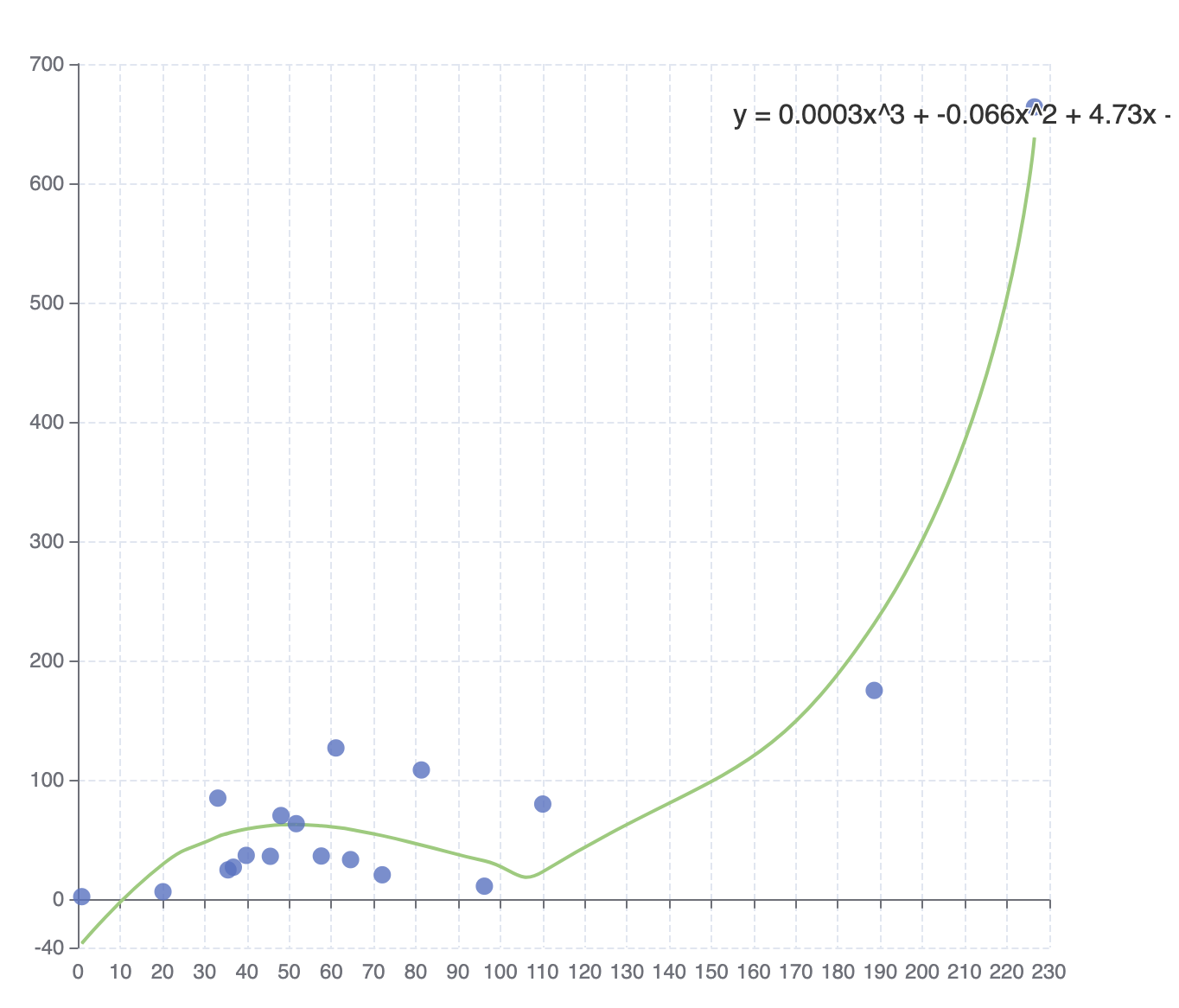Run

### Statistics

This interface provides basic summary statistical services.

#### ecStat.statistics.deviation()

##### Parameter
• `dataList` : `number[]`
##### Return Value
• `sampleDeviation`: `number`. Return the deviation of the numeric array dataList. If the dataList is empty or the length less than 2, return 0.

#### ecStat.statistics.sampleVariance()

##### Parameter
• `dataList` : `number[]`
##### Return Value
• `varianceValue`: `number`. Return the variance of the numeric array dataList. If the dataList is empty or the length less than 2, return 0.

#### ecStat.statistics.quantile()

##### Parameter
• `dataList` : `number[]`. Sorted array of numbers.
• `p`: `number`. where 0 =< p <= 1. For example, the first quartile at p = 0.25, the seconed quartile at p = 0.5(same as the median), and the third quartile at p = 0.75.
##### Return Value
• `quantileValue`: `number`. Return the quantile of the sorted array of numbers. If p <= 0 or the length of dataList less than 2, return the first element of the sorted array dataList; if p >= 1, return the last element of the sorted array dataList; If dataList is empty, return 0.

#### ecStat.statistics.max()

##### Parameter
• `dataList` : `number[]`
##### Return Value
• `maxValue`: `number`. The maximum value of the dataList.

#### ecStat.statistics.min()

##### Parameter
• `dataList` : `number[]`
##### Return Value
• `minValue`: `number`. The minimum value of the dataList.

#### ecStat.statistics.mean()

##### Parameter
• `dataList` : `number[]`
##### Return Value
• `meanValue`: `number`. The average of the dataList.

#### ecStat.statistics.median()

##### Parameter
• `dataList` : `number[]`. Sorted array of numbers
##### Return Value
• `medianValue`: `number`. The median of the dataList.

#### ecStat.statistics.sum()

##### Parameter
• `dataList` : `number[]`
##### Return Value
• `sumValue`: `number`. The sum of the dataList.

## Keywords

none

### Install

`npm i echarts-stat`

### Repository

github.com/ecomfe/echarts-stat

12,276

1.2.0